3.5 Family 1 of analysis : Compare different varieties evaluated for selection in different locations.

Family 1 gathers analyses that estimate entry effects. It allows to compare different entries on each location and test for significant differences among entries. Specific analysis including response to selection can also be done. The objective to compare different germplasms on each location for selection.

This can be done through (Figure 3.16) :

• classic anova (M4a, section 3.5.1) based on on fully replicated designs (D1, section 3.2.1),
• spatial analysis (M4b, section 3.5.2) based on row-column designs (D3, section 3.2.3),
• mixed models (M5, section3.5.3) for incomplete blocks designs (D2, section 3.2.2),
• bayesian hierarchical model intra-location (M7a, section 3.5.4) based on satellite-regional farms designs (D4, section 3.2.4).

It can be completed by organoleptic analysis (section 4). Based on these analysis, specific objective including response to selection analysis can also be done.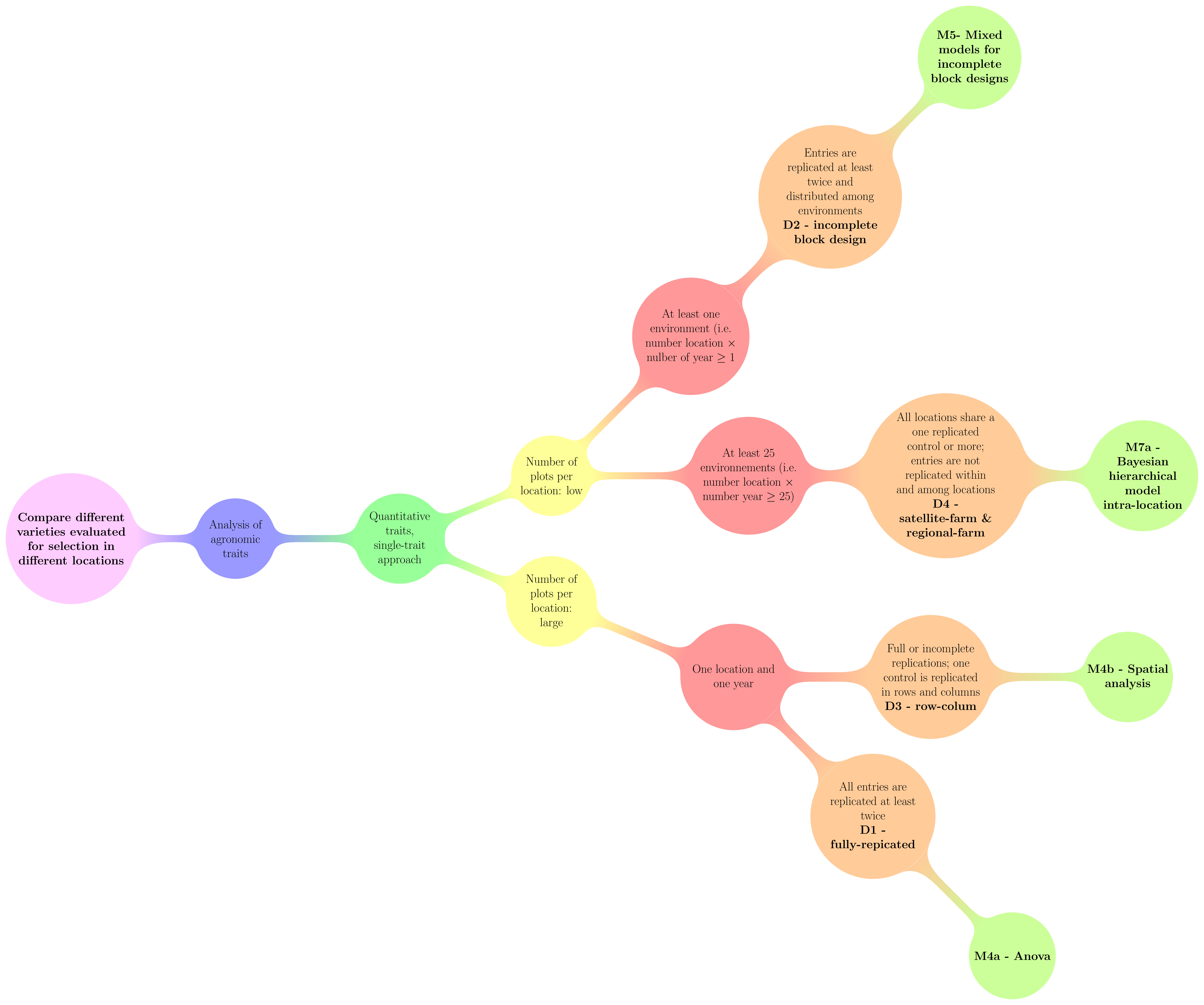Figure 3.16: Decision tree with experimental constraints, designs and methods of agronomic analysis carry out in PPBstats regarding the objective : Compare different varieties evaluated for selection in different locations. D refers to designs and M to methods.

3.5.1 Classic ANOVA (M4a)

!!! TO DO !!!

The model is based on frequentist statistics (section 3.1.2.1). The tests to check the model are explained in section 3.1.2.1.2. The method to compute mean comparison are explained in section 3.1.2.1.3.

3.5.2 Spatial analysis (M4b)

Note that this section is still under development : cf https://github.com/priviere/PPBstats/issues/20

3.5.2.1 Theory of the model

The experimental design used is the row-column design (D3). The following model is based on frequentist statistics (section 3.1.2.1). The model allows taking into account environmental variation within a block with few control replicated in rows and columns.

It is based on a SpATS (Spatial Analysis of Field Trials with Splines) model proposed by Rodríguez-Álvarez et al. (2016) :

$$Y_{ijk} = \alpha_{i} + r_{j} + c_{k} + f(u,v) + \varepsilon_{ijk}; \quad \varepsilon_{ijk} \sim \mathcal{N} (0,\sigma^2)$$

With,

• $$Y_{ijk}$$ the phenotypic value for germplasm $$i$$, row $$j$$ and column $$k$$
• $$\alpha_{i}$$ the effect of germplasm $$i$$
• $$r_{j}$$ the effect of row $$j$$
• $$c_{k}$$ the effect of col $$k$$
• $$f(u,v)$$ the smooth bivariate function that simultaneously accounts for the spatial trend across both directions of the fiel (i.e. rows and columns)
• $$\varepsilon_{ijk}$$ the residuals

Note that $$f(u,v)$$ is divided into 8 components excluding the intercept (Rodríguez-Álvarez et al. 2016):

• the linear effect of the rows (row),
• the linear effect of the columns (col),
• the linear interaction of rows and columns (row:col),
• the main row effect (f(row)),
• the main column effect (f(col)),
• the smooth varying coefficient term regarding rows (f(col):row),
• the smooth varying coefficient term regarding columns (row:f(col))),
• the smooth-by-smooth interaction component (f(col):f(row))

Much more information regarding the model as well as example of R package SpATS can be found in Rodríguez-Álvarez et al. (2016).

3.5.2.2 Steps with PPBstats

• Format the data with format_data_PPBstats()
• Run the model with model_spatial()
• Check model outputs with graphs to know if you can continue the analysis with check_model() and vizualise it with plot()
• Get mean comparisons for germplasms with mean_comparisons() and vizualise it with plot()

3.5.2.3 Format the data

p_case2 = design_experiment(
location = "Location-3",
year = "2016",
expe.type = "row-column",
germplasm = paste("germ", c(1:20), sep = ":"),
controls = "toto",
nb.controls.per.block = 7,
nb.blocks = 1,
nb.cols = 7)

d = p_case2$row-column$data.frame
d$variable = as.numeric(rnorm(nrow(d), 50, 10)) d = format_data_PPBstats(d, type = "data_agro") ## Warning in format_data_PPBstats.data_agro(data): Column "long" is needed to ## get map and not present in data. ## Warning in format_data_PPBstats.data_agro(data): Column "lat" is needed to ## get map and not present in data. ## data has been formated for PPBstats functions. 3.5.2.4 Run the model By default, germplasm are settled as random (genotype.as.random = TRUE). out_spatial = model_spatial(data = d, variable = "variable") ## Effective dimensions ## ------------------------- ## It. Deviance germplasm col_f row_f f(col) f(row) f(col):row col:f(row)f(col):f(row) ## 1 1348.286084 8.905 0.095 0.070 0.969 0.294 0.711 0.212 0.445 ## 2 134.600272 7.224 0.136 0.052 0.421 0.292 0.175 0.139 0.322 ## 3 133.596589 5.977 0.154 0.040 0.128 0.284 0.032 0.075 0.226 ## 4 133.166282 5.001 0.151 0.030 0.035 0.268 0.007 0.035 0.161 ## 5 132.923080 4.224 0.137 0.023 0.009 0.248 0.002 0.014 0.119 ## 6 132.761010 3.594 0.120 0.017 0.002 0.226 0.000 0.005 0.092 ## 7 132.642980 3.077 0.102 0.013 0.001 0.205 0.000 0.002 0.072 ## 8 132.552667 2.646 0.085 0.010 0.000 0.186 0.000 0.001 0.059 ## 9 132.481577 2.285 0.070 0.008 0.000 0.169 0.000 0.000 0.048 ## 10 132.424653 1.980 0.056 0.006 0.000 0.154 0.000 0.000 0.041 ## 11 132.378553 1.722 0.045 0.004 0.000 0.141 0.000 0.000 0.035 ## 12 132.340908 1.501 0.036 0.003 0.000 0.129 0.000 0.000 0.030 ## 13 132.309956 1.312 0.028 0.003 0.000 0.119 0.000 0.000 0.026 ## 14 132.284351 1.150 0.022 0.002 0.000 0.110 0.000 0.000 0.023 ## 15 132.263049 1.010 0.017 0.002 0.000 0.102 0.000 0.000 0.021 ## 16 132.245231 0.890 0.013 0.001 0.000 0.095 0.000 0.000 0.018 ## 17 132.230249 0.785 0.010 0.001 0.000 0.089 0.000 0.000 0.017 ## 18 132.217586 0.694 0.008 0.001 0.000 0.083 0.000 0.000 0.015 ## 19 132.206831 0.614 0.006 0.001 0.000 0.078 0.000 0.000 0.014 ## 20 132.197654 0.545 0.005 0.001 0.000 0.073 0.000 0.000 0.012 ## 21 132.189788 0.484 0.003 0.000 0.000 0.069 0.000 0.000 0.011 ## 22 132.183016 0.430 0.003 0.000 0.000 0.065 0.000 0.000 0.010 ## 23 132.177163 0.383 0.002 0.000 0.000 0.062 0.000 0.000 0.010 ## 24 132.172084 0.341 0.002 0.000 0.000 0.059 0.000 0.000 0.009 ## 25 132.167663 0.305 0.001 0.000 0.000 0.056 0.000 0.000 0.008 ## 26 132.163801 0.272 0.001 0.000 0.000 0.053 0.000 0.000 0.008 ## 27 132.160417 0.243 0.001 0.000 0.000 0.051 0.000 0.000 0.007 ## 28 132.157444 0.217 0.000 0.000 0.000 0.048 0.000 0.000 0.007 ## 29 132.154826 0.195 0.000 0.000 0.000 0.046 0.000 0.000 0.006 ## 30 132.152514 0.174 0.000 0.000 0.000 0.044 0.000 0.000 0.006 ## 31 132.150468 0.156 0.000 0.000 0.000 0.043 0.000 0.000 0.005 ## 32 132.148654 0.140 0.000 0.000 0.000 0.041 0.000 0.000 0.005 ## 33 132.147044 0.126 0.000 0.000 0.000 0.039 0.000 0.000 0.005 ## 34 132.145611 0.113 0.000 0.000 0.000 0.038 0.000 0.000 0.004 ## 35 132.144335 0.101 0.000 0.000 0.000 0.036 0.000 0.000 0.004 ## 36 132.143196 0.091 0.000 0.000 0.000 0.035 0.000 0.000 0.004 ## 37 132.142180 0.082 0.000 0.000 0.000 0.034 0.000 0.000 0.004 ## 38 132.141270 0.073 0.000 0.000 0.000 0.033 0.000 0.000 0.003 ## Timings: ## SpATS 0.61 seconds ## All process 0.756 seconds ## ## Spatial analysis of trials with splines ## ## Response: variable ## Genotypes (as random): germplasm ## Spatial: ~PSANOVA(col, row, nseg = c(nlevels(data_tmp$X), nlevels(data_tmp$Y))) ## Random: ~col_f + row_f ## ## ## Number of observations: 28 ## Number of missing data: 0 ## Effective dimension: 4.11 ## Deviance: 132.141 ## ## Variance components: ## Variance SD log10(lambda) ## germplasm 3.070e-01 5.541e-01 2.49352 ## col_f 1.292e-04 1.137e-02 5.86952 ## row_f 6.877e-05 8.293e-03 6.14329 ## f(col) 2.024e-21 4.499e-11 22.67436 ## f(row) 4.720e+00 2.173e+00 1.30670 ## f(col):row 1.869e-19 4.324e-10 20.70898 ## col:f(row) 3.992e-17 6.318e-09 18.37950 ## f(col):f(row) 3.077e-01 5.547e-01 2.49255 ## ## Residual 9.565e+01 9.780e+00 ## ## Spatial analysis of trials with splines ## ## Response: variable ## Genotypes (as random): germplasm ## Spatial: ~PSANOVA(col, row, nseg = c(nlevels(data_tmp$X), nlevels(data_tmp$Y))) ## Random: ~col_f + row_f ## ## ## Number of observations: 28 ## Number of missing data: 0 ## Effective dimension: 4.11 ## Deviance: 132.141 ## ## Dimensions: ## Effective Model Nominal Ratio Type ## Intercept 1.0 1 1 1.00 F ## germplasm 0.1 22 21 0.00 R ## col_f 0.0 7 6 0.00 R ## row_f 0.0 4 3 0.00 R ## col 1.0 1 1 1.00 S ## row 1.0 1 1 1.00 S ## rowcol 1.0 1 1 1.00 S ## f(col) 0.0 8 8 0.00 S ## f(row) 0.0 5 5 0.01 S ## f(col):row 0.0 8 8 0.00 S ## col:f(row) 0.0 5 5 0.00 S ## f(col):f(row) 0.0 40 40 0.00 S ## ## Total 4.1 103 100 0.04 ## Residual 23.9 ## Nobs 28 ## ## Type codes: F 'Fixed' R 'Random' S 'Smooth/Semiparametric' out_spatial is a list containing two elements : • info : a list with variable and data out_spatial$info$variable ##  "variable" head(out_spatial$info$data) ## location year germplasm block X Y variable ## 1 Location-3 2016 germ:5 1 A 1 61.20858 ## 2 Location-3 2016 germ:9 1 A 2 41.49280 ## 3 Location-3 2016 toto 1 A 3 46.89400 ## 4 Location-3 2016 XXX-1 1 A 4 54.24332 ## 5 Location-3 2016 germ:14 1 B 1 43.28034 ## 6 Location-3 2016 toto 1 B 2 37.33528 • model a list with five elements : • model out_spatial$model$model ## ## Spatial analysis of trials with splines ## ## Response: variable ## Genotypes (as random): germplasm ## Spatial: ~PSANOVA(col, row, nseg = c(nlevels(data_tmp$X), nlevels(data_tmp$Y))) ## Random: ~col_f + row_f ## ## ## Number of observations: 28 ## Number of missing data: 0 ## Effective dimension: 4.11 ## Deviance: 132.141 • summary out_spatial$model$summary ## ## Spatial analysis of trials with splines ## ## Response: variable ## Genotypes (as random): germplasm ## Spatial: ~PSANOVA(col, row, nseg = c(nlevels(data_tmp$X), nlevels(data_tmp$Y))) ## Random: ~col_f + row_f ## ## ## Number of observations: 28 ## Number of missing data: 0 ## Effective dimension: 4.11 ## Deviance: 132.141 ## ## Dimensions: ## Effective Model Nominal Ratio Type ## Intercept 1.0 1 1 1.00 F ## germplasm 0.1 22 21 0.00 R ## col_f 0.0 7 6 0.00 R ## row_f 0.0 4 3 0.00 R ## col 1.0 1 1 1.00 S ## row 1.0 1 1 1.00 S ## rowcol 1.0 1 1 1.00 S ## f(col) 0.0 8 8 0.00 S ## f(row) 0.0 5 5 0.01 S ## f(col):row 0.0 8 8 0.00 S ## col:f(row) 0.0 5 5 0.00 S ## f(col):f(row) 0.0 40 40 0.00 S ## ## Total 4.1 103 100 0.04 ## Residual 23.9 ## Nobs 28 ## ## Type codes: F 'Fixed' R 'Random' S 'Smooth/Semiparametric' • germplasm_effects out_spatial$model$germplasm_effects ## toto germ:11 germ:4 germ:9 germ:6 germ:14 germ:1 germ:7 ## 50.05549 50.06708 50.08321 50.08324 50.08455 50.09559 50.10104 50.10172 ## germ:17 germ:16 germ:3 XXX-1 germ:13 germ:20 germ:19 germ:15 ## 50.10399 50.10790 50.11185 50.11282 50.12276 50.12624 50.13590 50.14377 ## germ:12 germ:2 germ:5 germ:18 germ:10 germ:8 ## 50.14403 50.14674 50.15140 50.15688 50.15884 50.16194 • var_res the variance of the residuals out_spatial$model$var_res ##  95.64558 3.5.2.5 Check and visualize model outputs The tests to check the model are explained in section 3.1.2.1.2. 3.5.2.5.1 Check the model out_check_spatial = check_model(out_spatial) out_check_spatial is a list containing two elements : • spatial the output from the model • data_ggplot a list containing information for ggplot: • data_ggplot_residuals a list containing : • data_ggplot_normality • data_ggplot_skewness_test • data_ggplot_kurtosis_test • data_ggplot_qqplot • data_ggplot_variability_repartition_pie 3.5.2.5.2 Visualize outputs Once the computation is done, you can visualize the results with plot p_out_check_spatial = plot(out_check_spatial) p_out_check_spatial is a list with: • residuals • histogram : histogram with the distribution of the residuals p_out_check_spatial$residuals$histogram ## stat_bin() using bins = 30. Pick better value with binwidth.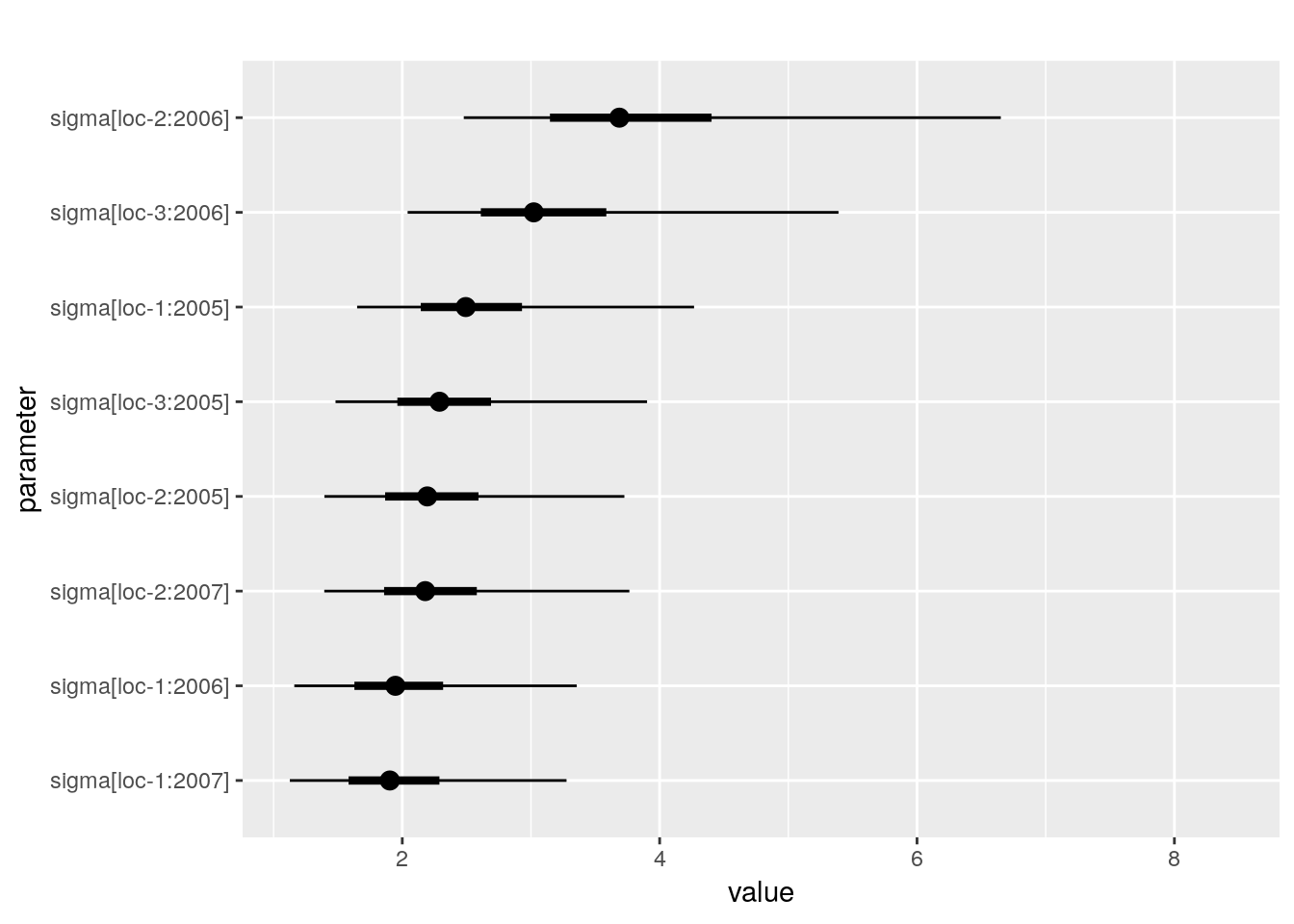• qqplot p_out_check_spatial$residuals$qqplot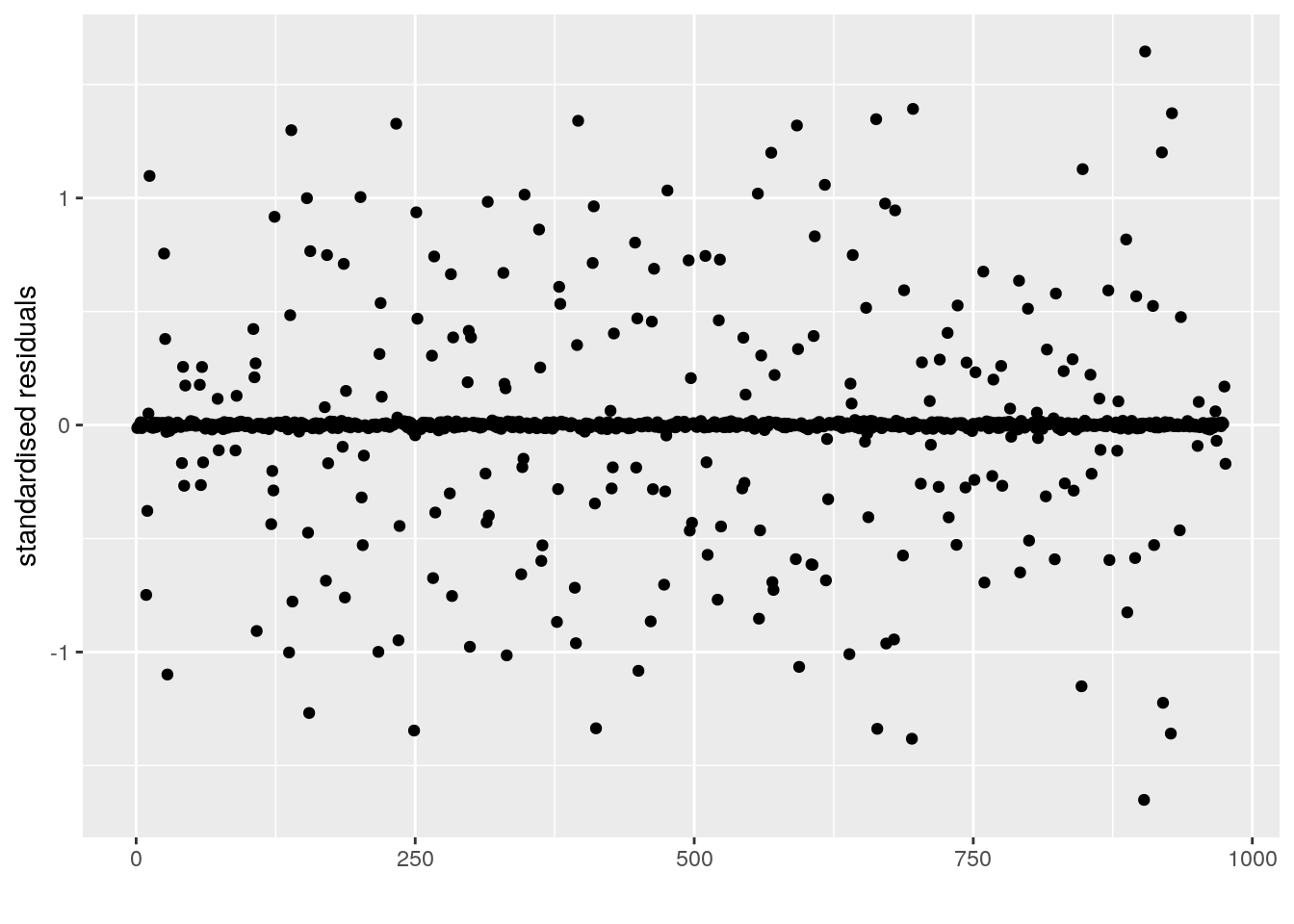• variability_repartition : pie with repartition of variance for each factor p_out_check_spatial$variability_repartition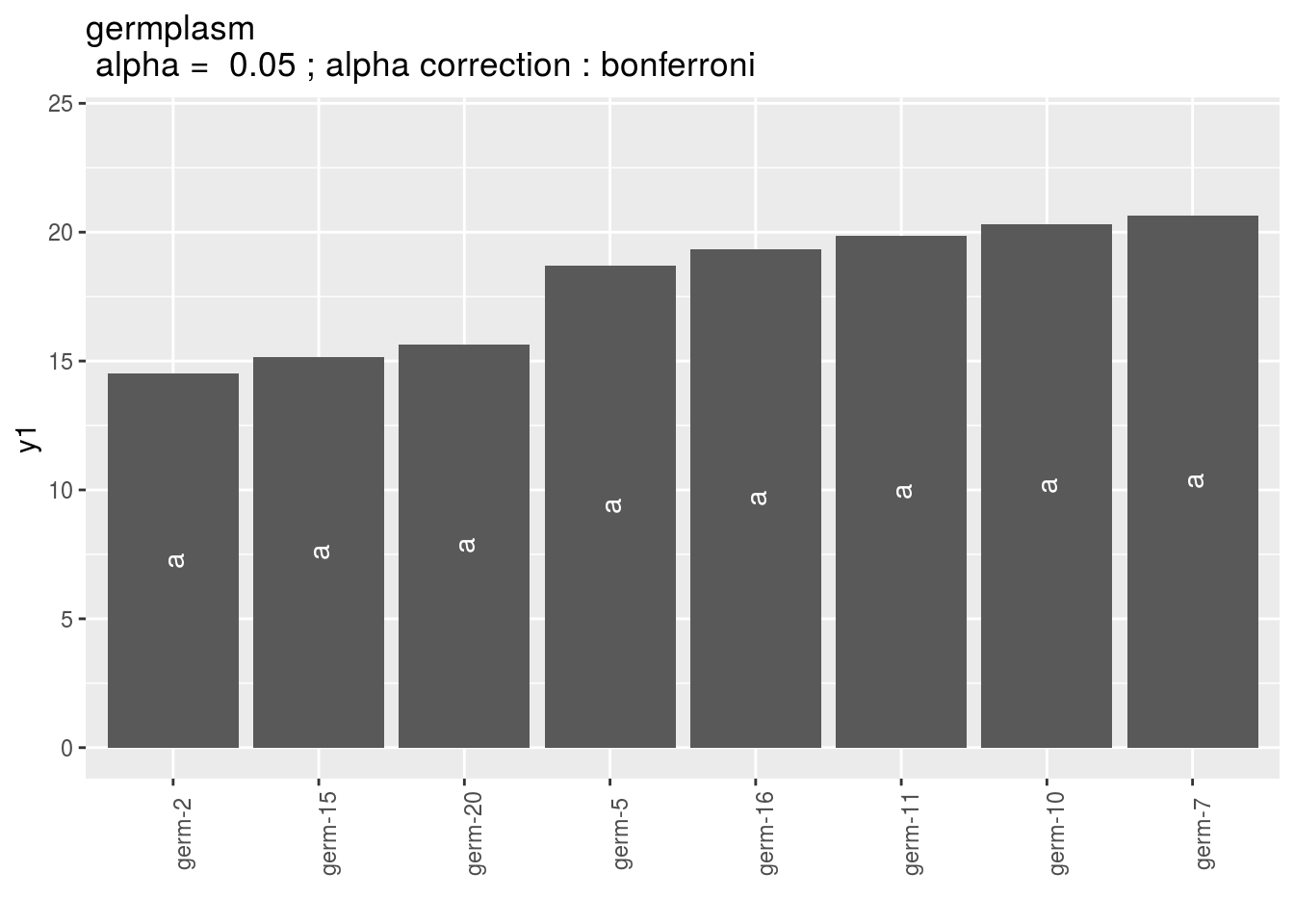3.5.2.6 Get and visualize mean comparisons

The method to compute mean comparison are explained in section 3.1.2.1.3.

3.5.2.7 Get mean comparisons

Get mean comparisons with mean_comparisons.

out_mean_comparisons_spatial = mean_comparisons(out_check_spatial, p.adj = "bonferroni")

out_mean_comparisons_spatial is a list of two elements:

• info : a list with variable and data
• data_ggplot_LSDbarplot_germplasm

3.5.2.8 Visualize mean comparisons

p_out_mean_comparisons_spatial = plot(out_mean_comparisons_spatial)

p_out_mean_comparisons_spatial is a list of one elements with barplots :

For each element of the list, there are as many graph as needed with nb_parameters_per_plot parameters per graph. Letters are displayed on each bar. Parameters that do not share the same letters are different regarding type I error (alpha) and alpha correction. The error I (alpha) and the alpha correction are displayed in the title.

germplasm : mean comparison for germplasm

pg = p_out_mean_comparisons_spatial$germplasm names(pg) ##  "1" "2" "3" pg$1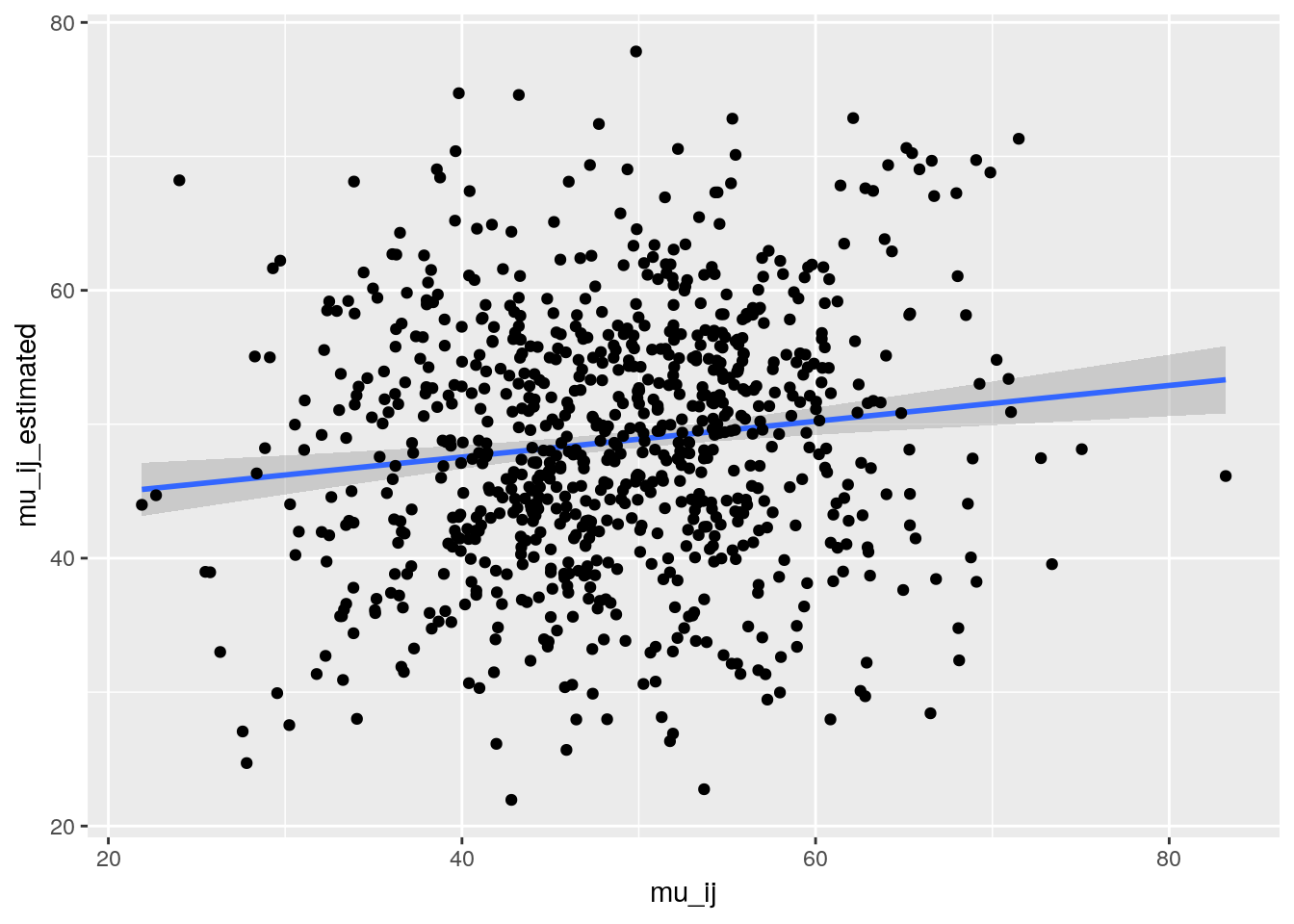3.5.2.9 Apply the workflow to several variables

If you wish to apply the spatial workflow to several variables, you can use lapply with the following code :

workflow_spatial = function(x){
out_spatial = model_spatial(data = d, variable = "variable")

out_check_spatial = check_model(out_spatial)
p_out_check_spatial = plot(out_check_spatial)

out_mean_comparisons_spatial = mean_comparisons(out_check_spatial, p.adj = "bonferroni")
p_out_mean_comparisons_spatial = plot(out_mean_comparisons_spatial)

out = list(
out_spatial = out_spatial,
out_check_spatial = out_check_spatial,
p_out_check_spatial = p_out_check_spatial,
out_mean_comparisons_spatial = out_mean_comparisons_spatial,
p_out_mean_comparisons_spatial = p_out_mean_comparisons_spatial
)
return(out)
}

## Not run
# vec_variables = c("y1", "y2", "y3")
#
# out = lapply(vec_variables, workflow_spatial)
# names(out) = vec_variables

3.5.3 Mixted model for incomplete block design (M5)

!!! TO DO !!!

The model is based on frequentist statistics (section 3.1.2.1). The tests to check the model are explained in section 3.1.2.1.2. The method to compute mean comparison are explained in section 3.1.2.1.3.

#library(ibd)
#data(ibddata)
#aov.ibd(y~factor(trt)+factor(blk),data=ibddata)
#contrast=matrix(c(1,-1,0,0,0,0,0,0,0,0,0,1,-1,0,0,0,0,0),nrow=2,byrow=TRUE)
#aov.ibd(y~factor(trt)+factor(blk),specs="trt",data=ibddata,contrast=contrast)

3.5.4 Hierarchical Bayesian intra-location model to perform mean comparisons with each farm (M7a)

At the farm level, the residual has few degrees of freedom, leading to a poor estimation of the residual variance and to a lack of power for comparing populations. model_bh_intra_location was implemented to improve efficiency of mean comparisons. It is efficient with more than 20 environment (i.e. location $$\times$$ year) (Rivière et al. 2015). The model is based on bayesian statistics (section 3.1.2.2).

3.5.4.1 Theory of the model

The experimental design used is satellite and regional farms (D4). The model is described in Rivière et al. (2015). We restricted ourselves to analysing values at the plot level (the values may result from the average of individual plants measures). The phenotypic value $$Y_{ijk}$$ for variable $$Y$$, germplasm $$i$$, environment $$j$$ and block $$k$$ is modelled as :

$$Y_{ijk} = \mu_{ij} + \beta_{jk} + \varepsilon_{ijk} ; \quad \varepsilon_{ijk} \sim \mathcal{N} (0,\sigma^2_{j})$$,

where

• $$\mu_{ij}$$ is the mean of germplasm $$i$$ in environment $$j$$ (note that this parameter, which corresponds to an entry, confounds the population effect and the population $$\times$$ environment effect);
• $$\beta_{jk}$$ is the effect of block $$k$$ in environment $$j$$ satisfying the constraint6 $$\sum\limits_{k=1}^K \beta_{jk} = 1$$ ;
• $$\varepsilon_{ijk}$$ is the residual error;
• $$\mathcal{N} (0,\sigma^2_{j})$$ denotes normal distribution centred on 0 with variance $$\sigma^2_{j}$$, which is specific to environment $$j$$.

We take advantage of the similar structure of the trials in each environment of the network to assume that trial residual variances come from a common distribution :

$$\sigma^2_{j} \sim \frac{1}{Gamma(\nu,\rho)}$$,

where $$\nu$$ and $$\rho$$ are unknown parameters. Because of the low number of residual degrees of freedom for each farm, we use a hierarchical approach in order to assess mean differences on farm. For that, we place vague prior distributions on the hyperparameters $$\nu$$ and $$\rho$$ :

$$\nu \sim Uniform(\nu_{min},\nu_{max}) ; \quad \rho \sim Gamma(10^{-6},10^{-6})$$.

In other words, the residual variance of a trial in a given environment is estimated using all the informations available on the network rather than using the data from that particular trial only.

The parameters $$\mu_{ij}$$ and $$\beta_{jk}$$ are assumed to follow vague prior distributions too:

$$\mu_{ij} \sim \mathcal{N}(\mu_{.j},10^{6}); \quad \beta_{jk} \sim \mathcal{N}(0,10^{6})$$.

The inverse gamma distribution has a minimum value of 0 (consistent with the definition of a variance) and may have various shapes including asymmetric distributions. From an agronomical point of view, the assumption that trial variances are heterogeneous is consistent with organic farming: there are as many environments as farms and farmers leading to a high heterogeneity. Environment is here considered in a broad sense: practices (sowing date, sowing density, tilling, etc.), pedo climatic conditions, biotic and abiotic stress, … (Desclaux et al. 2008). Moreover, the inverse gamma distribution has conjugate properties that facilitate MCMC convergence. This model is therefore a good choice based on both agronomic and statistical criteria.

The residual variance estimated from the controls is assumed to be representative of the residual variance of the other entries. Blocks are included in the model only if the trial has blocks.

3.5.4.2 Steps with

To run model_bh_intra_location, follow these steps (Figure 3.2):

• Format the data with format_data_PPBstats()
• Run the model with model_bh_intra_location()
• Check model outputs with graphs using check_model()to know if you can continue the analysis
• Get mean comparisons for each factor with mean_comparisons() and vizualise it with plot()

3.5.4.3 Format the data

The values for $$\mu_{ij}$$, $$\beta_{jk}$$, $$\epsilon_{ijk}$$ and $$\sigma_j$$ are the real value used to create the simulated dataset. This dataset is representative of data obtain in a PPB programme.

data(data_model_bh_intra_location)
data_model_bh_intra_location = format_data_PPBstats(data_model_bh_intra_location, type = "data_agro")
## Warning in format_data_PPBstats.data_agro(data): Column "long" is needed to
## get map and not present in data.
## Warning in format_data_PPBstats.data_agro(data): Column "lat" is needed to
## get map and not present in data.
## data has been formated for PPBstats functions.
head(data_model_bh_intra_location) # first rows of the data
##   location year germplasm block X Y      tkw   tkw$date mu_ij beta_jk ## 1 loc-1 2005 germ-25 1 1 a 72.09900 2017-07-22 73.37224 0 ## 2 loc-1 2005 germ-26 1 2 b 61.05274 2017-07-22 61.61823 0 ## 3 loc-1 2005 germ-27 1 3 c 62.99350 2017-07-22 64.31830 0 ## 4 loc-1 2005 germ-28 1 4 d 65.10909 2017-07-22 62.57840 0 ## 5 loc-1 2005 germ-25 2 5 e 77.01361 2017-07-22 73.37224 0 ## 6 loc-1 2005 germ-26 2 6 f 64.10541 2017-07-22 61.61823 0 ## epsilon_ijk sigma_j tkw$date_julian
## 1  -1.2732421 1.622339             202
## 2  -0.5654918 1.622339             202
## 3  -1.3248006 1.622339             202
## 4   2.5306946 1.622339             202
## 5   3.6413666 1.622339             202
## 6   2.4871807 1.622339             202

3.5.4.4 Run the model

To run model model_bh_intra_location on the dataset, used the function model_bh_intra_location(). Here it is run on thousand kernel weight (tkw).

By default, model_bh_intra_location returns posteriors for $$\mu_{ij}$$ (return.mu = TRUE), $$\beta_{jk}$$ (return.beta = TRUE), $$\sigma_j$$ (return.sigma = TRUE), $$\nu$$ (return.nu = TRUE) and $$\rho$$ (return.rho = TRUE). You can also get $$\epsilon_{ijk}$$ value with return.espilon = TRUE.

DIC criterion is a generalization of the AIC criterion that can be used for hierarchical models (Spiegelhalter et al. 2002). The smaller the DIC value, the better the model (Plummer 2008). By default, DIC is not displayed, you can ask for this value to compare to other model (DIC = TRUE).

# out_model_bh_intra_location = model_bh_intra_location(data = data_model_bh_intra_location, variable = "tkw", return.epsilon = TRUE)

# Compiling model graph
# Resolving undeclared variables
# Allocating nodes
# Graph information:
#   Observed stochastic nodes: 976
# Unobserved stochastic nodes: 927
# Total graph size: 8609
#
# Initializing model
#
# |++++++++++++++++++++++++++++++++++++++++++++++++++| 100%
# |**************************************************| 100%
# |**************************************************| 100%
# |**************************************************| 100%

load("./data_PPBstats/out_model_bh_intra_location.RData") # To save time

You can get information on the environments in the dataset :

out_model_bh_intra_location$vec_env_with_no_data ## NULL out_model_bh_intra_location$vec_env_with_no_controls
##  "loc-25:2005"
out_model_bh_intra_location$vec_env_with_controls ##  "loc-10:2005" "loc-10:2006" "loc-10:2007" "loc-11:2005" "loc-11:2006" ##  "loc-11:2007" "loc-1:2005" "loc-1:2006" "loc-1:2007" "loc-12:2005" ##  "loc-12:2006" "loc-12:2007" "loc-13:2005" "loc-13:2006" "loc-13:2007" ##  "loc-14:2005" "loc-14:2006" "loc-14:2007" "loc-15:2005" "loc-15:2006" ##  "loc-15:2007" "loc-16:2005" "loc-16:2006" "loc-16:2007" "loc-17:2005" ##  "loc-17:2006" "loc-17:2007" "loc-18:2005" "loc-18:2006" "loc-18:2007" ##  "loc-19:2006" "loc-19:2007" "loc-20:2006" "loc-20:2007" "loc-21:2006" ##  "loc-21:2007" "loc-2:2005" "loc-2:2006" "loc-2:2007" "loc-22:2006" ##  "loc-22:2007" "loc-23:2006" "loc-23:2007" "loc-3:2005" "loc-3:2006" ##  "loc-3:2007" "loc-4:2005" "loc-4:2006" "loc-4:2007" "loc-5:2006" ##  "loc-6:2006" "loc-6:2007" "loc-7:2006" "loc-7:2007" "loc-8:2006" ##  "loc-9:2005" "loc-9:2006" "loc-9:2007" out_model_bh_intra_location$vec_env_RF
##   "loc-1:2005" "loc-1:2006" "loc-1:2007" "loc-2:2005" "loc-2:2006"
##   "loc-2:2007" "loc-3:2005" "loc-3:2006" "loc-3:2007" "loc-4:2005"
##  "loc-4:2006" "loc-4:2007" "loc-5:2006" "loc-6:2006" "loc-6:2007"
##  "loc-7:2006" "loc-7:2007" "loc-8:2006"
out_model_bh_intra_location$vec_env_SF ##  "loc-10:2005" "loc-10:2006" "loc-10:2007" "loc-11:2005" "loc-11:2006" ##  "loc-11:2007" "loc-12:2005" "loc-12:2006" "loc-12:2007" "loc-13:2005" ##  "loc-13:2006" "loc-13:2007" "loc-14:2005" "loc-14:2006" "loc-14:2007" ##  "loc-15:2005" "loc-15:2006" "loc-15:2007" "loc-16:2005" "loc-16:2006" ##  "loc-16:2007" "loc-17:2005" "loc-17:2006" "loc-17:2007" "loc-18:2005" ##  "loc-18:2006" "loc-18:2007" "loc-19:2006" "loc-19:2007" "loc-20:2006" ##  "loc-20:2007" "loc-21:2006" "loc-21:2007" "loc-22:2006" "loc-22:2007" ##  "loc-23:2006" "loc-23:2007" "loc-9:2005" "loc-9:2006" "loc-9:2007" Below is an example with low nb_iterations (see section 3.1.2.2 about the number of iterations): # out_model_bh_intra_location_bis = model_bh_intra_location(data = data_model_bh_intra_location, variable = "tkw", nb_iteration = 5000) # Compiling model graph # Resolving undeclared variables # Allocating nodes # Graph information: # Observed stochastic nodes: 976 # Unobserved stochastic nodes: 927 # Total graph size: 8609 # # Initializing model # # |++++++++++++++++++++++++++++++++++++++++++++++++++| 100% # |**************************************************| 100% # |**************************************************| 100% # Warning message: # In model_bh_intra_location(data = data_model_bh_intra_location, variable = "tkw", nb_iteration = 5000) : # nb_iterations is below 20 000, which seems small to get convergence in the MCMC. load("./data_PPBstats/out_model_bh_intra_location_bis.RData") # To save time 3.5.4.5 Check and visualize model outputs The tests to check the model are explained in section 3.1.2.2.2. 3.5.4.5.1 Check the model Once the model has been run, it is necessary to check if the outputs can be taken with confidence. This step is needed before going ahead in the analysis (in fact the object used in the next functions must come from check_model). # out_check_model_bh_intra_location = check_model(out_model_bh_intra_location) # The Gelman-Rubin test is running for each parameter ... # The two MCMC for each parameter converge thanks to the Gelman-Rubin test. load("./data_PPBstats/out_check_model_bh_intra_location.RData") # To save time out_check_model_bh_intra_location is a list containing: • MCMC : a data fame resulting from the concatenation of the two MCMC for each parameter. This object can be used for further analysis. There are as many columns as parameters and as many rows as iterations/thin (the thin value is 10 by default in the models). dim(out_check_model_bh_intra_location$MCMC)
##  20000   945
• MCMC_conv_not_ok: a data fame resulting from the concatenation of the two MCMC for each parameter for environments where some parameters did not converge for mu and beta

• data_env_with_no_controls : data frame with environnements without controls

• data_env_whose_param_did_not_converge : a list with data frame with environments where some parameters did not converge for mu and beta

• data_ggplot : a list containing information for ggplot:
• sigma_j
• mu_ij
• beta_jk
• sigma_j_2
• epsilon_ijk

When considering out_model_bh_intra_location_bis:

# out_check_model_bh_intra_location_bis = check_model(out_model_bh_intra_location_bis)

# The Gelman-Rubin test is running for each parameter ...
# The two MCMC of the following parameters do not converge thanks to the Gelman-Rubin test : # mu[germ-20,loc-15:2006], nu, rho, sigma[loc-15:2006]. Therefore, they are not present in MCMC output.
# MCMC are updated, the following environment were deleted : loc-15:2006
# data_env_whose_param_did_not_converge contains the raw data for these environments.

load("./data_PPBstats/out_check_model_bh_intra_location_bis.RData") # To save time
3.5.4.5.2 Visualize outputs

Once the computation is finished, you can visualize the results with

p_out_check_model_bh_intra_location = plot(out_check_model_bh_intra_location)
## Distribution of sigma_j in the inverse Gamme distribution are done.
## The mu_ij posterior distributions are done.
## The beta_jk posterior distributions are done.
## The sigma_j posterior distributions are done.
## The standardised residuals distributions are done.

p_out_check_model_bh_intra_location is a list with:

• sigma_j_gamma : mean of each sigma_j displayed on the Inverse Gamma distribution. The first graph represents all the sigma_j, the other graph represent the same information divided into several graphs (based on argument nb_parameters_per_plot).
p_out_check_model_bh_intra_location$sigma_j_gamma[]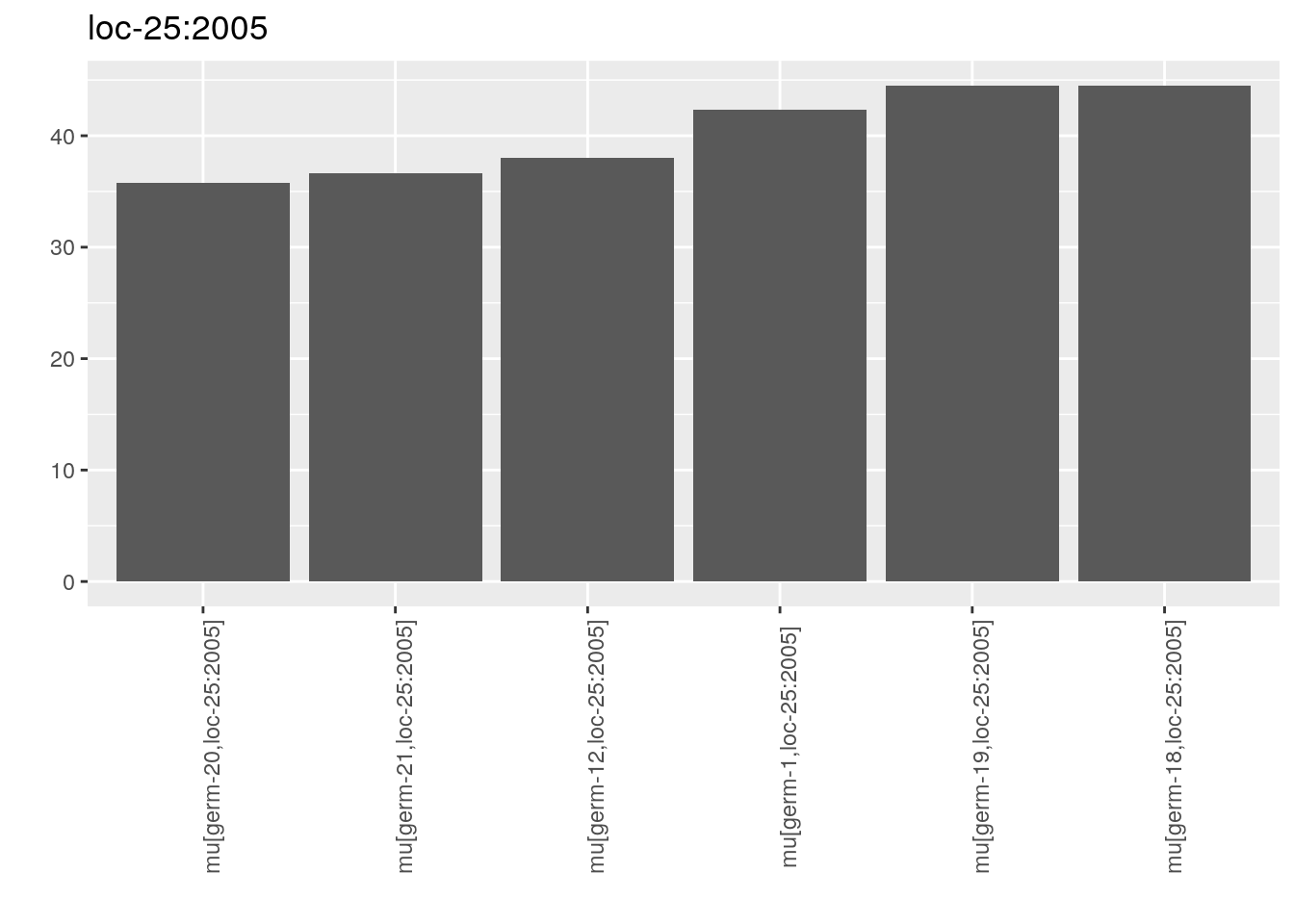p_out_check_model_bh_intra_location$sigma_j_gamma[]• mu_ij : distribution of each mu_ij in a list with as many elements as environment. For each element of the list, there are as many graph as needed with nb_parameters_per_plot mu_ij per graph.
names(p_out_check_model_bh_intra_location$mu_ij) ##  "loc-10:2005" "loc-10:2006" "loc-10:2007" "loc-11:2005" "loc-11:2006" ##  "loc-11:2007" "loc-1:2005" "loc-1:2006" "loc-1:2007" "loc-12:2005" ##  "loc-12:2006" "loc-12:2007" "loc-13:2005" "loc-13:2006" "loc-13:2007" ##  "loc-14:2005" "loc-14:2006" "loc-14:2007" "loc-15:2005" "loc-15:2006" ##  "loc-15:2007" "loc-16:2005" "loc-16:2006" "loc-16:2007" "loc-17:2005" ##  "loc-17:2006" "loc-17:2007" "loc-18:2005" "loc-18:2006" "loc-18:2007" ##  "loc-19:2006" "loc-19:2007" "loc-20:2006" "loc-20:2007" "loc-21:2006" ##  "loc-21:2007" "loc-2:2005" "loc-2:2006" "loc-2:2007" "loc-22:2006" ##  "loc-22:2007" "loc-23:2006" "loc-23:2007" "loc-3:2005" "loc-3:2006" ##  "loc-3:2007" "loc-4:2005" "loc-4:2006" "loc-4:2007" "loc-5:2006" ##  "loc-6:2006" "loc-6:2007" "loc-7:2006" "loc-7:2007" "loc-8:2006" ##  "loc-9:2005" "loc-9:2006" "loc-9:2007" names(p_out_check_model_bh_intra_location$mu_ij$loc-10:2005) ##  "1" p_out_check_model_bh_intra_location$mu_ij$loc-10:2005$1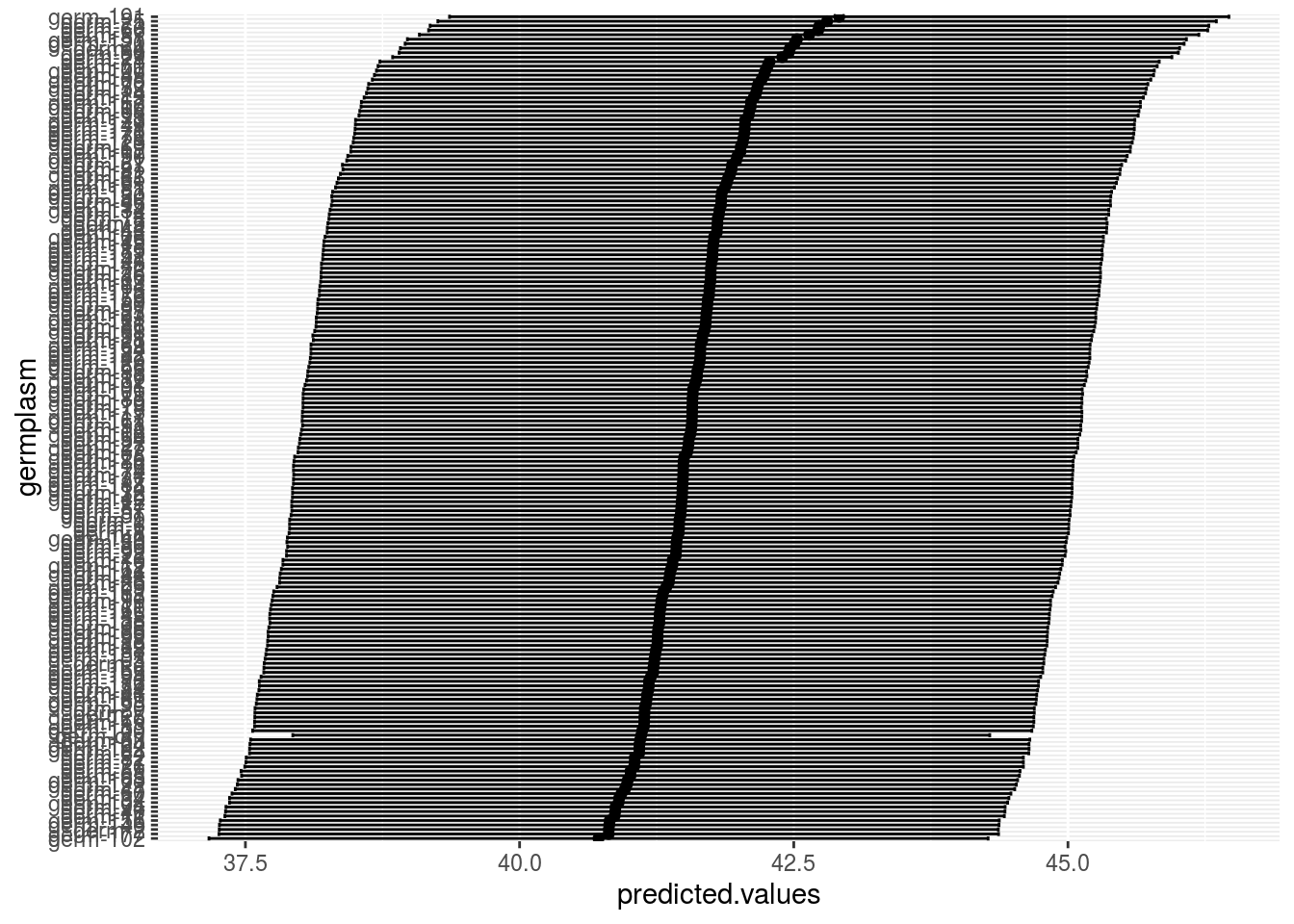• beta_jk : distribution of each beta_jk in a list with as many elements as environment. For each element of the list, there are as many graph as needed with nb_parameters_per_plot beta_jk per graph.
names(p_out_check_model_bh_intra_location$beta_jk) ##  "loc-1:2005" "loc-1:2006" "loc-1:2007" "loc-2:2005" "loc-2:2006" ##  "loc-2:2007" "loc-3:2005" "loc-3:2006" "loc-3:2007" "loc-4:2005" ##  "loc-4:2006" "loc-4:2007" "loc-5:2006" "loc-6:2006" "loc-6:2007" ##  "loc-7:2006" "loc-7:2007" "loc-8:2006" names(p_out_check_model_bh_intra_location$beta_jk$loc-1:2005) ##  "1" p_out_check_model_bh_intra_location$beta_jk$loc-1:2005$1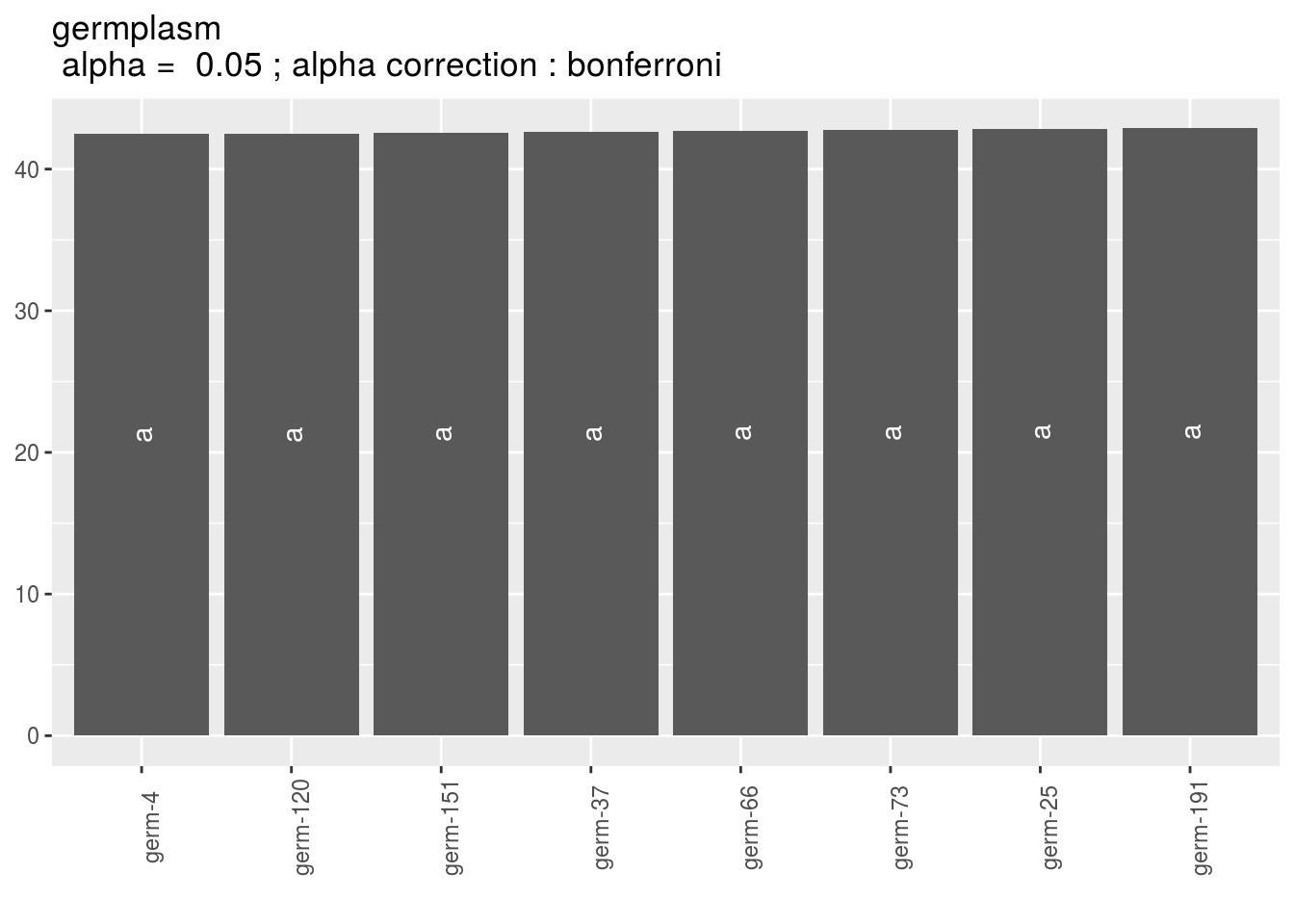• sigma_j : distribution of each sigma_j. There are as many graph as needed with nb_parameters_per_plot sigma_j per graph.
names(p_out_check_model_bh_intra_location$sigma_j) ##  "1" "2" "3" "4" "5" "6" "7" "8" p_out_check_model_bh_intra_location$sigma_j[]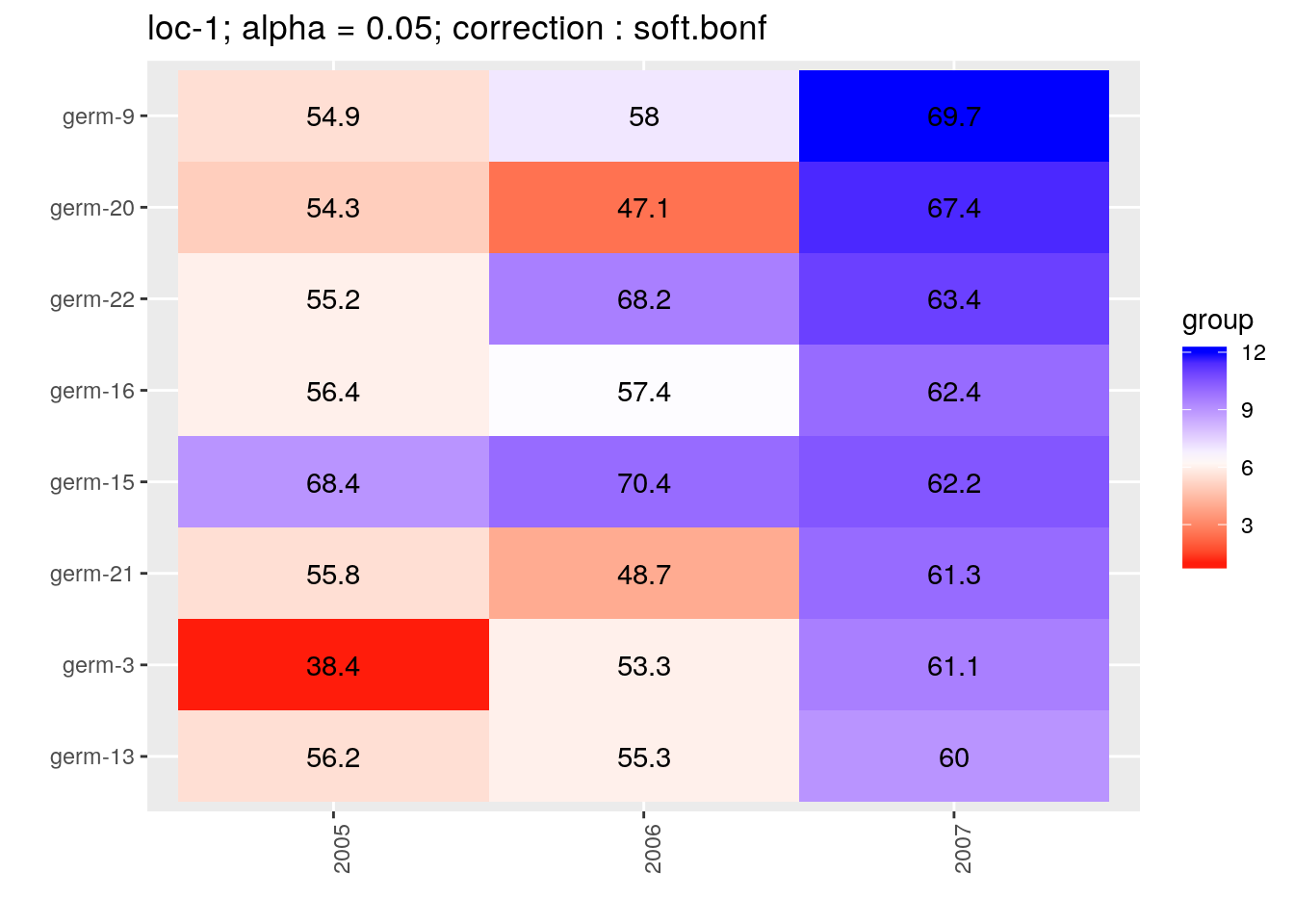• epsilon_ijk : standardised residuals distribution. If the model went well it should be between -2 and 2.
p_out_check_model_bh_intra_location$epsilon_ijk• mcmc_not_converge_traceplot_density : a list with the plots of trace and density to check the convergence of the two MCMC only for chains that are not converging detected by the Gelman-Rubin test. If all the chains converge, it is NULL p_out_check_model_bh_intra_location$mcmc_not_converge_traceplot_density
## NULL

Here all the parameters converged.

When considering p_out_check_model_bh_intra_location_bis, there is no convergence because the MCMC are too small.

p_out_check_model_bh_intra_location_bis = plot(out_check_model_bh_intra_location_bis)
## Distribution of sigma_j in the inverse Gamme distribution are done.
## The mu_ij posterior distributions are done.
## The beta_jk posterior distributions are done.
## The sigma_j posterior distributions are done.
## Trace and density plot for MCMC that did not converged are done.
p_out_check_model_bh_intra_location_bis$mcmc_not_converge_traceplot_density$sigma\$loc-15:2006 ## traceplot## ## densityJust for fun, you can compare the posterior medians and the arithmetic means for the mu_ij. MCMC = out_check_model_bh_intra_locationMCMC effects = apply(MCMC, 2, median) mu_ij_estimated = effects[grep("mu",names(effects))] names(mu_ij_estimated) = sapply(names(mu_ij_estimated), function(x){ sub("\$", "", sub("mu\\[", "", x)) }
)

d = filter(data_model_bh_intra_location, location != "loc-24")
d = filter(d, location != "loc-25")
d = droplevels(d)
environment = paste(as.character(d$location), as.character(d$year), sep = ":")
d$entry = as.factor(paste(as.character(d$germplasm), environment, sep = ","))
mu_ij = tapply(d$mu_ij, d$entry, mean, na.rm = TRUE)

check_data = cbind.data.frame(mu_ij, mu_ij_estimated[names(mu_ij)])

Let’s have a look on the relation between the posterior medians and the arithmetic means. It goes pretty well!

p = ggplot(check_data, aes(x = mu_ij, y = mu_ij_estimated))
p + stat_smooth(method = "lm") + geom_point()3.5.4.6 Get and visualize mean comparisons

The method to compute mean comparison are explained in section ??.

3.5.4.6.1 Get mean comparisons

Get mean comparisons with mean_comparisons. The theory behind mean comparisons has been explained in section ??.

Below is an example for $$\mu$$, the same can be done for $$\beta$$.

# out_mean_comparisons_model_bh_intra_location_mu = mean_comparisons(out_check_model_bh_intra_location, parameter = "mu")

# Get at least X groups for loc-11:2007. It may take some time ...
# Get at least X groups for loc-11:2007 is done.
# Get at least X groups for loc-17:2005. It may take some time ...
# Get at least X groups for loc-17:2005 is done.
# Get at least X groups for loc-21:2006. It may take some time ...
# Get at least X groups for loc-21:2006 is done.
# Get at least X groups for loc-9:2006. It may take some time ...
# Get at least X groups for loc-9:2006 is done.

load("./data_PPBstats/out_mean_comparisons_model_bh_intra_location_mu.RData") # To save time

out_mean_comparisons_model_bh_intra_location_mu is a list of three elements:

• data_mean_comparisons a list with as many elements as environment.
head(names(out_mean_comparisons_model_bh_intra_location_mu$data_mean_comparisons)) ##  "loc-1:2005" "loc-1:2006" "loc-1:2007" "loc-2:2005" "loc-2:2006" ##  "loc-2:2007" Each element of the list is composed of two elements: - mean.comparisons r head(out_mean_comparisons_model_bh_intra_location_mu$data_mean_comparisons$loc-1:2005$mean.comparisons)



##                parameter   median groups nb_group alpha alpha.correction
## 1  mu[germ-3,loc-1:2005] 38.42457      a       10  0.05        soft.bonf
## 2  mu[germ-1,loc-1:2005] 41.73724     ab       10  0.05        soft.bonf
## 3 mu[germ-10,loc-1:2005] 45.91424    abc       10  0.05        soft.bonf
## 4 mu[germ-18,loc-1:2005] 47.76006   abcd       10  0.05        soft.bonf
## 5  mu[germ-2,loc-1:2005] 48.10288  abcde       10  0.05        soft.bonf
## 6 mu[germ-19,loc-1:2005] 49.20361 abcdef       10  0.05        soft.bonf
##     entry environment location year
## 1  germ-3  loc-1:2005    loc-1 2005
## 2  germ-1  loc-1:2005    loc-1 2005
## 3 germ-10  loc-1:2005    loc-1 2005
## 4 germ-18  loc-1:2005    loc-1 2005
## 5  germ-2  loc-1:2005    loc-1 2005
## 6 germ-19  loc-1:2005    loc-1 2005


- Mpvalue : a square matrix with pvalue computed for each pair of parameter.

r
out_mean_comparisons_model_bh_intra_location_mu$data_mean_comparisons$loc-1:2005$Mpvalue[1:3, 1:3]   ## mu[germ-3,loc-1:2005] mu[germ-1,loc-1:2005] ## mu[germ-3,loc-1:2005] 0 0.17505 ## mu[germ-1,loc-1:2005] 0 0.00000 ## mu[germ-10,loc-1:2005] 0 0.00000 ## mu[germ-10,loc-1:2005] ## mu[germ-3,loc-1:2005] 0.03940 ## mu[germ-1,loc-1:2005] 0.15185 ## mu[germ-10,loc-1:2005] 0.00000  • data_env_with_no_controls a list with as many elements as environment. names(out_mean_comparisons_model_bh_intra_location_mu$data_env_with_no_controls)
##  "loc-25:2005"

Each list contains mean.comparisons. Note there are no groups displayed. Inded, there were no controls on the environment so no model was run.

head(out_mean_comparisons_model_bh_intra_location_mu$data_env_with_no_controls$loc-25:2005$mean.comparisons) ## entry germplasm environment block X Y median ## 1 germ-12,loc-25:2005 germ-12 loc-25:2005 1 4 d 38.02544 ## 2 germ-18,loc-25:2005 germ-18 loc-25:2005 1 5 e 44.49495 ## 3 germ-19,loc-25:2005 germ-19 loc-25:2005 1 6 f 44.46948 ## 4 germ-1,loc-25:2005 germ-1 loc-25:2005 1 3 c 42.32750 ## 5 germ-20,loc-25:2005 germ-20 loc-25:2005 1 7 g 35.77590 ## 6 germ-21,loc-25:2005 germ-21 loc-25:2005 1 8 h 36.59313 ## parameter location year ## 1 mu[germ-12,loc-25:2005] loc-25 2005 ## 2 mu[germ-18,loc-25:2005] loc-25 2005 ## 3 mu[germ-19,loc-25:2005] loc-25 2005 ## 4 mu[germ-1,loc-25:2005] loc-25 2005 ## 5 mu[germ-20,loc-25:2005] loc-25 2005 ## 6 mu[germ-21,loc-25:2005] loc-25 2005 • data_env_whose_param_did_not_converge a list with as many elements as environment. names(out_mean_comparisons_model_bh_intra_location_mu$data_env_whose_param_did_not_converge)
## NULL

Here it is NULL as all parameters converge. Otherwise in each list it is mean.comparisons. Note there are no groups displayed. Inded, the model did not well so no mean comparisons were done.

head(out_mean_comparisons_model_bh_intra_location_mu$data_env_with_no_controls$loc-5:2005$mean.comparisons) ## NULL 3.5.4.6.2 Visualize mean comparisons To see the output, use plot. On each plot, the alpha (type one error) value and the alpha correction are displayed. alpha = Imp means that no differences could be detected. For plot_type = "interaction" and plot_type = "score", it is displayed under the form: alpha | alpha correction. The ggplot are done for each element of the list coming from mean_comparisons. For each plot_type, it is a list of three lists each with as many elements as environments. For each element of the list, there are as many graphs as needed with nb_parameters_per_plot parameters per graph. 3.5.4.6.2.1 barplot p_barplot_mu = plot(out_mean_comparisons_model_bh_intra_location_mu, plot_type = "barplot") names(p_barplot_mu) ##  "data_mean_comparisons" ##  "data_env_with_no_controls" ##  "data_env_whose_param_did_not_converge" From data_mean_comparisons, only environments where all MCMC converged are represented. Letters are displayed on each bar. Parameters that do not share the same letters are different based on type I error (alpha) and alpha correction. The error I (alpha) and the alpha correction are displayed in the title. alpha = Imp means that no significant differences coumd be detected. p = p_barplot_mu$data_mean_comparisons

head(names(p))
##  "loc-1:2005" "loc-1:2006" "loc-1:2007" "loc-2:2005" "loc-2:2006"
##  "loc-2:2007"
p_env = p$loc-1:2005 names(p_env) ##  "1" "2" "3" "4" p_env$1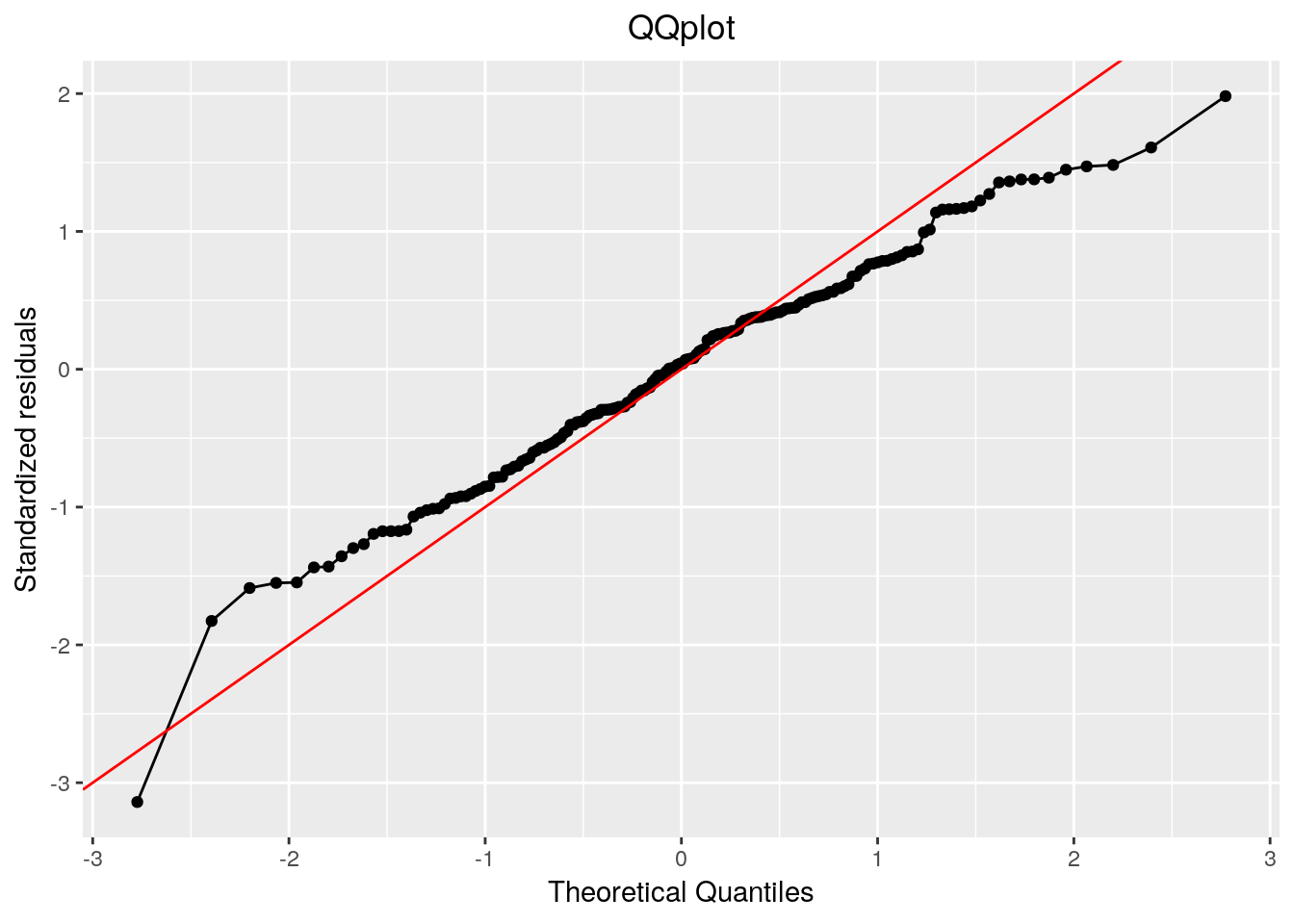p_env$2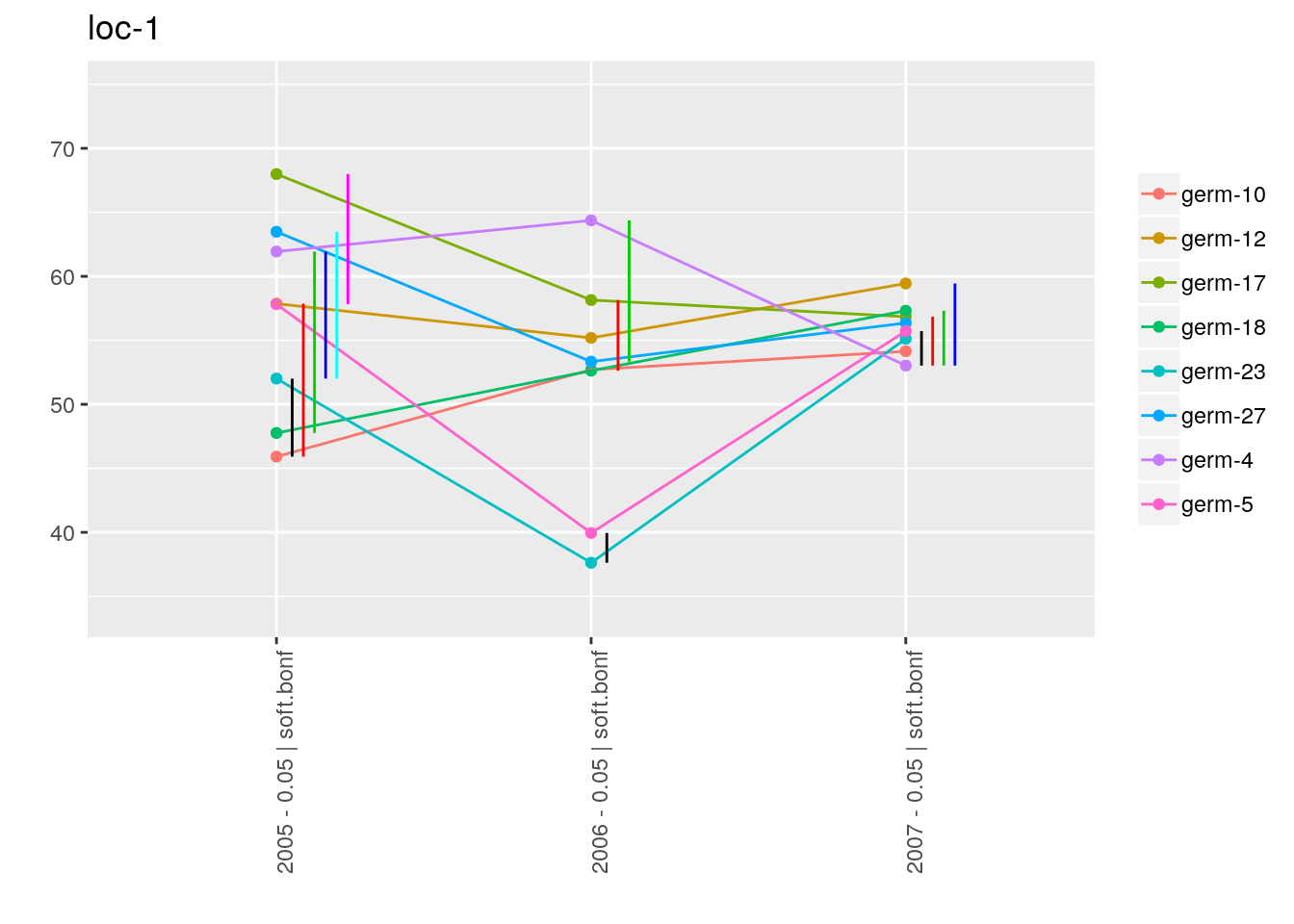p_env$3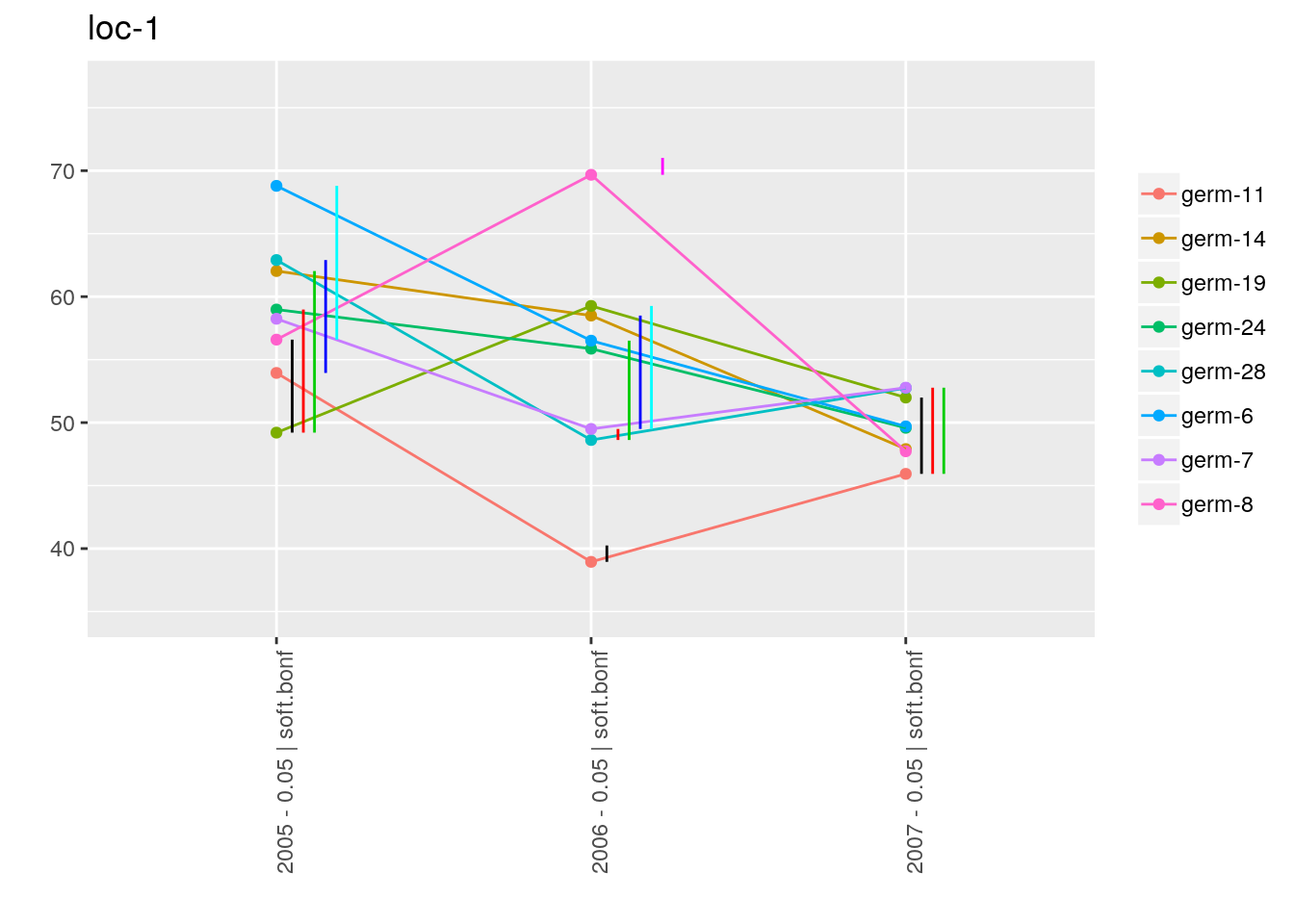p_env$4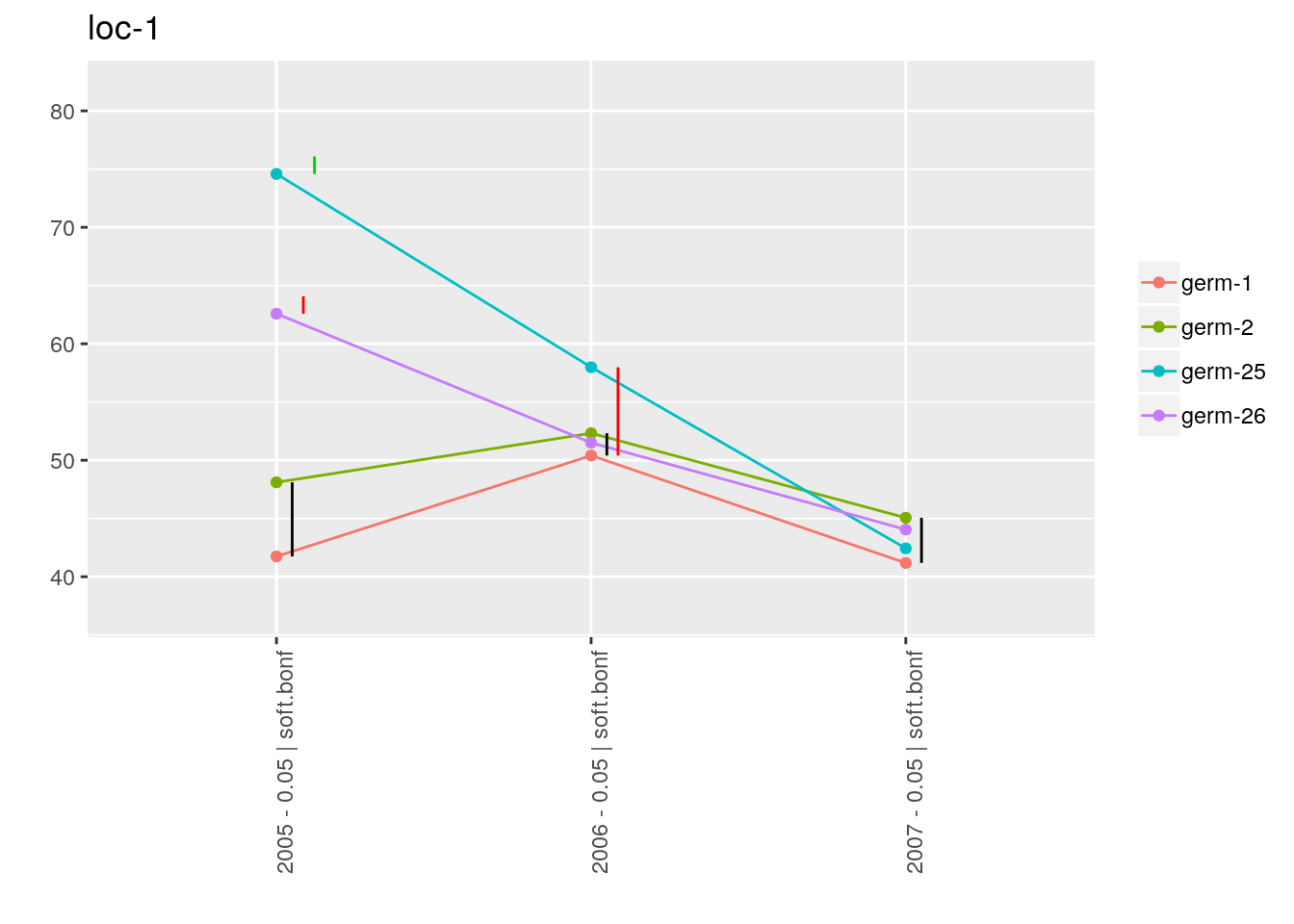For data_env_with_no_controls, only environments where there were no controls are represented. p_barplot_mu$data_env_with_no_controls
## $loc-25:2005 ##$loc-25:2005$1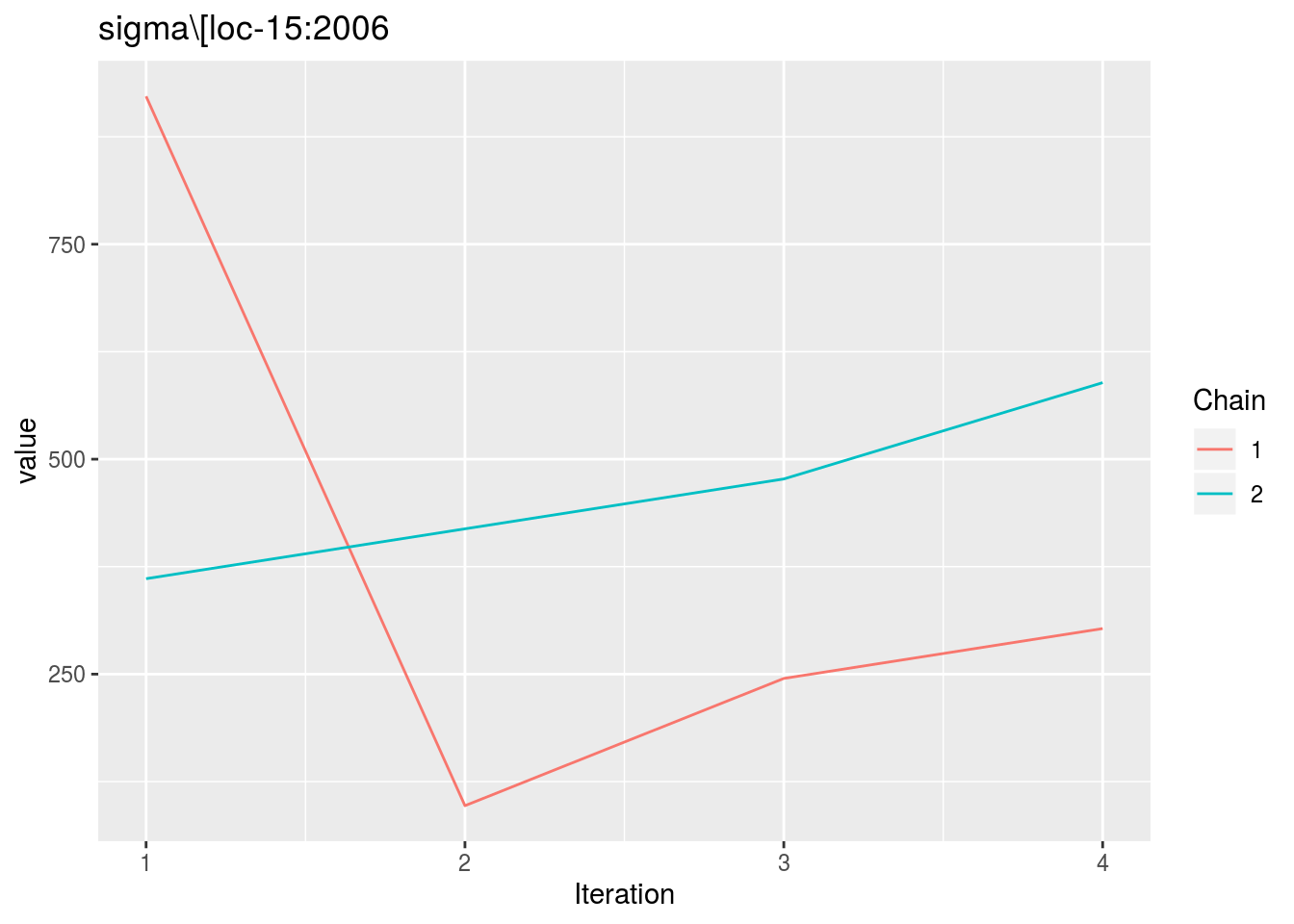For data_env_whose_param_did_not_converge, only environments where MCMC did not converge are represented. p_barplot_mu$data_env_whose_param_did_not_converge
## list()
3.5.4.6.2.2 interaction

With plot_type = "interaction", you can display the year effect as well as detect groups. One group is represented by one vertical line. Germplasms which share the same group are not different. Germplasms which do not share the same groupe are different (Section ??).

The ggplot are done for each element of the list coming rom mean_comparisons.

For each plot_type, it is a list of three elements being lists with as many elements as environment.

For each element of the list, there are as many graph as needed with nb_parameters_per_plot parameters per graph.

p_interaction = plot(out_mean_comparisons_model_bh_intra_location_mu, plot_type = "interaction")

head(names(p_interaction$data_mean_comparisons)) ##  "loc-1" "loc-10" "loc-11" "loc-12" "loc-13" "loc-14" p_env = p_interaction$data_mean_comparisons$loc-1 names(p_env) ##  "1" "2" "3" "4" p_env$1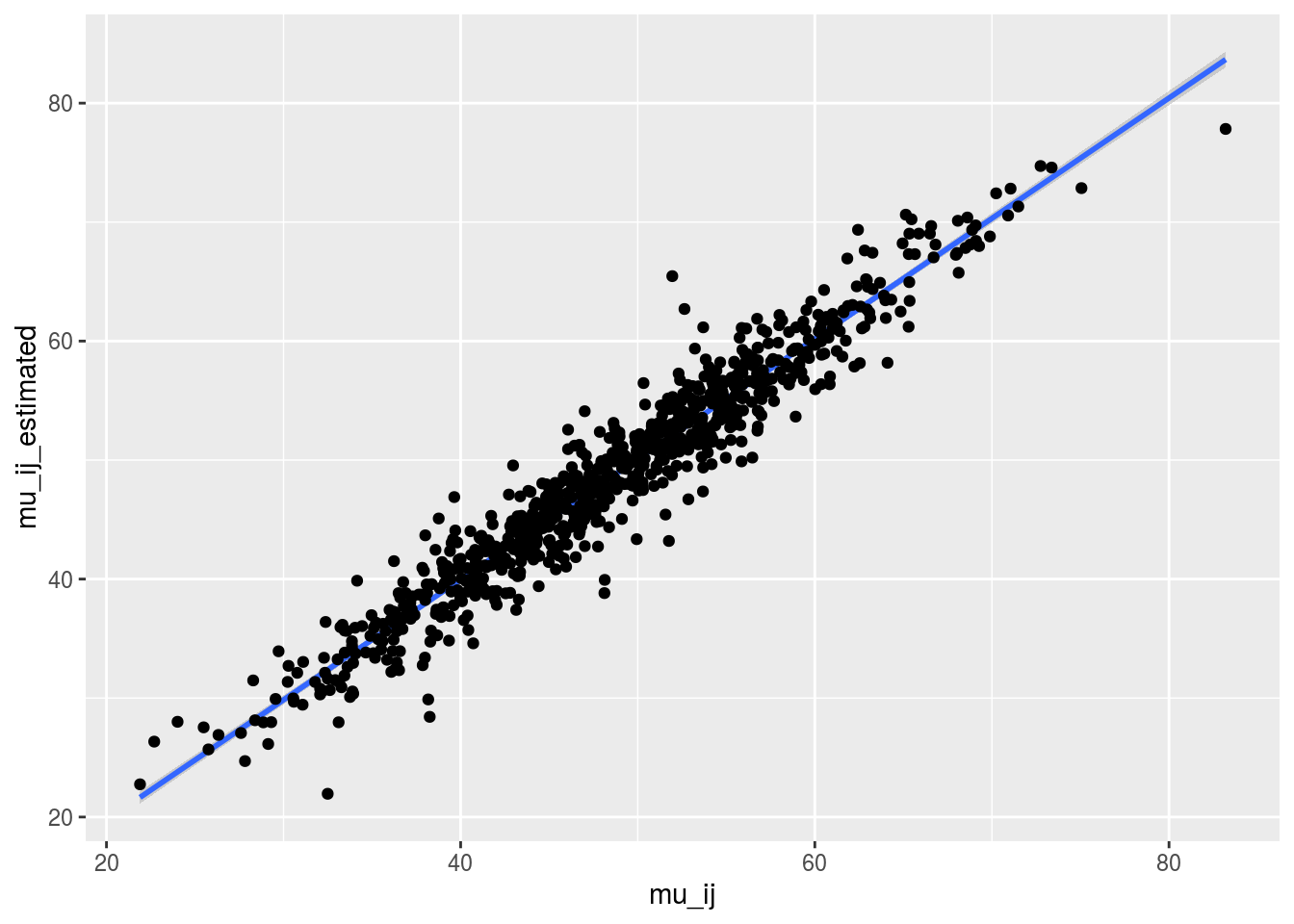p_env$2p_env$3p_env$43.5.4.6.3 score For the score, more entries are displayed. An high score means that the entry was in a group with an high mean. A low socre means that the entry was in a group with an low mean. In the legend, the score goes from 1 (first group) to the number of groups of significativity. This plot is useful to look at year effects. p_score = plot(out_mean_comparisons_model_bh_intra_location_mu, plot_type = "score") head(names(p_score)) ##  "loc-1" "loc-10" "loc-11" "loc-12" "loc-13" "loc-14" p_env = p_score$loc-1
names(p_env)
##  "1" "2" "3" "4"
p_env$1p_env$2p_env$3p_env$43.5.4.7 Apply the workflow to several variables

If you wish to apply the model_bh_intra_location workflow to several variables, you can use lapply with the following code :

workflow_model_bh_intra_location = function(x){
out_model_bh_intra_location = model_bh_intra_location(data = data_model_bh_intra_location, variable = x, return.epsilon = TRUE)

out_check_model_bh_intra_location = check_model(out_model_bh_intra_location)
p_out_check_model_bh_intra_location = plot(out_check_model_bh_intra_location)

out_mean_comparisons_model_bh_intra_location_mu = mean_comparisons(out_check_model_bh_intra_location, parameter = "mu")
p_barplot_mu = plot(out_mean_comparisons_model_bh_intra_location_mu, plot_type = "barplot")
p_interaction = plot(out_mean_comparisons_model_bh_intra_location_mu, plot_type = "interaction")
p_score = plot(out_mean_comparisons_model_bh_intra_location_mu, plot_type = "score")

out = list(
"out_model_bh_intra_location" = out_model_bh_intra_location,
"out_check_model_bh_intra_location" = out_check_model_bh_intra_location,
"p_out_check_model_bh_intra_location" = p_out_check_model_bh_intra_location,
"out_mean_comparisons_model_bh_intra_location_mu" = out_mean_comparisons_model_bh_intra_location_mu,
"p_barplot_mu" = p_barplot_mu,
"p_interaction" = p_interaction,
"p_score" = p_score
)
return(out)
}

## Not run because of memory and time issues !
# vec_variables = c("y1", "y2", "y3")
#
# out = lapply(vec_variables, workflow_model_bh_intra_location)
# names(out) = vec_variables

References

Rodríguez-Álvarez, X.M., M. P. Boer, F. A. van Eeuwijk, and P. H. C. Eilers. 2016. “Spatial Models for Field Trials.” ArXiv E-Prints, July.

Rivière, P., J.C. Dawson, I. Goldringer, and O. David. 2015. “Hierarchical Bayesian Modeling for Flexible Experiments in Decentralized Participatory Plant Breeding.” Crop Science 55 (3).

Desclaux, D., J. M. Nolot, Y. Chiffoleau, C. Leclerc, and E. Gozé. 2008. “Changes in the Concept of Genotype X Environment Interactions to Fit Agriculture Diversification and Decentralized Participatory Plant Breeding: Pluridisciplinary Point of View.” Euphytica 163: 533–46.

Spiegelhalter, D. J., N. G. Best, B. P. Carlin, and A. Van Der Linde. 2002. “Bayesian Measures of Model Complexity and Fit.” Journal of the Royal Statistical Society: Series B (Statistical Methodology) 64 (4): 583–639.

Plummer, M. 2008. “Penalized Loss Functions for Bayesian Model Comparison.” Biostatistics 9 (3): 523–39.

1. Note that it is quite different from Rivière et al. (2015) where the model was written only for two blocks. Here there is no restriction on the number of blocks.